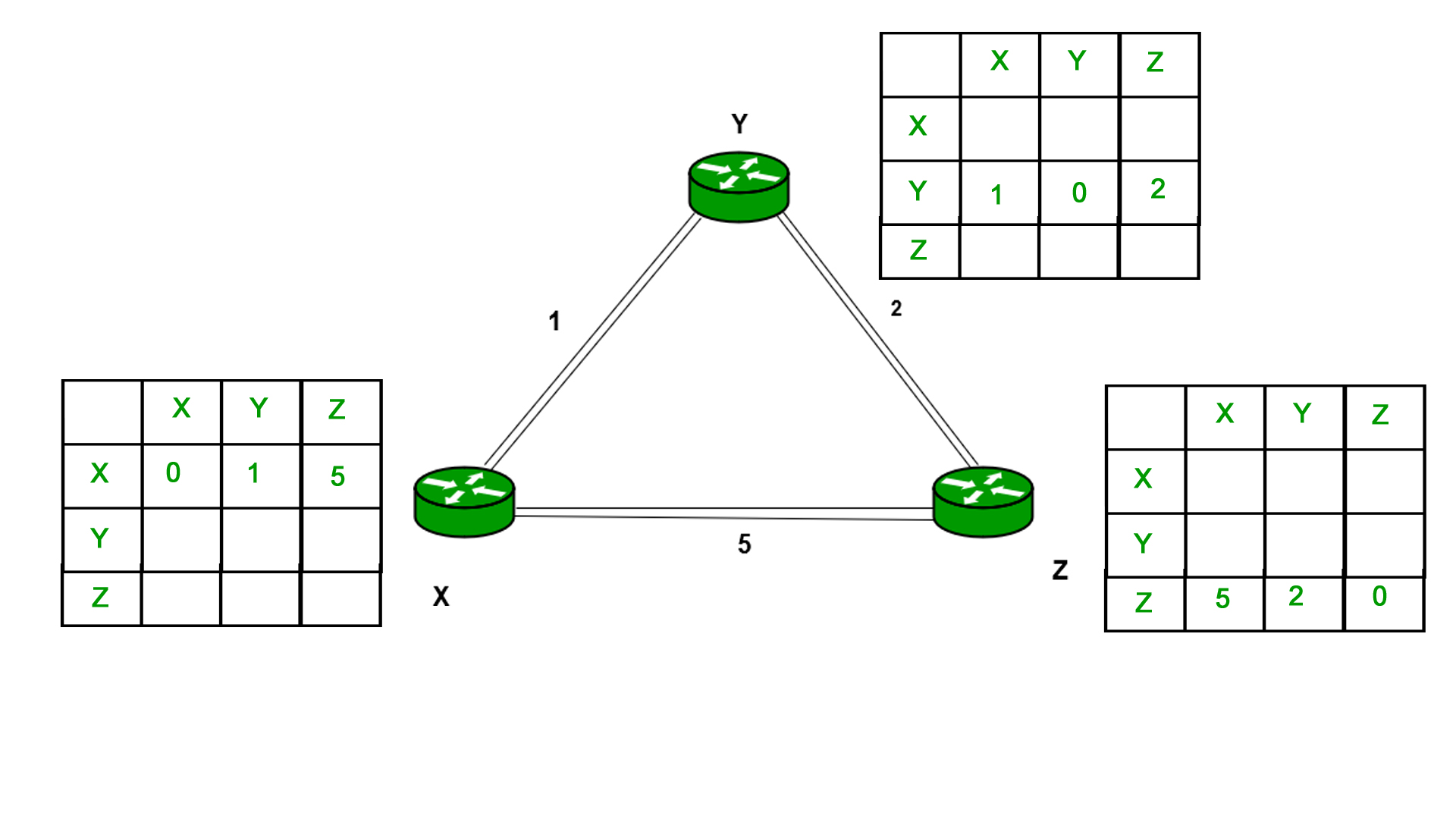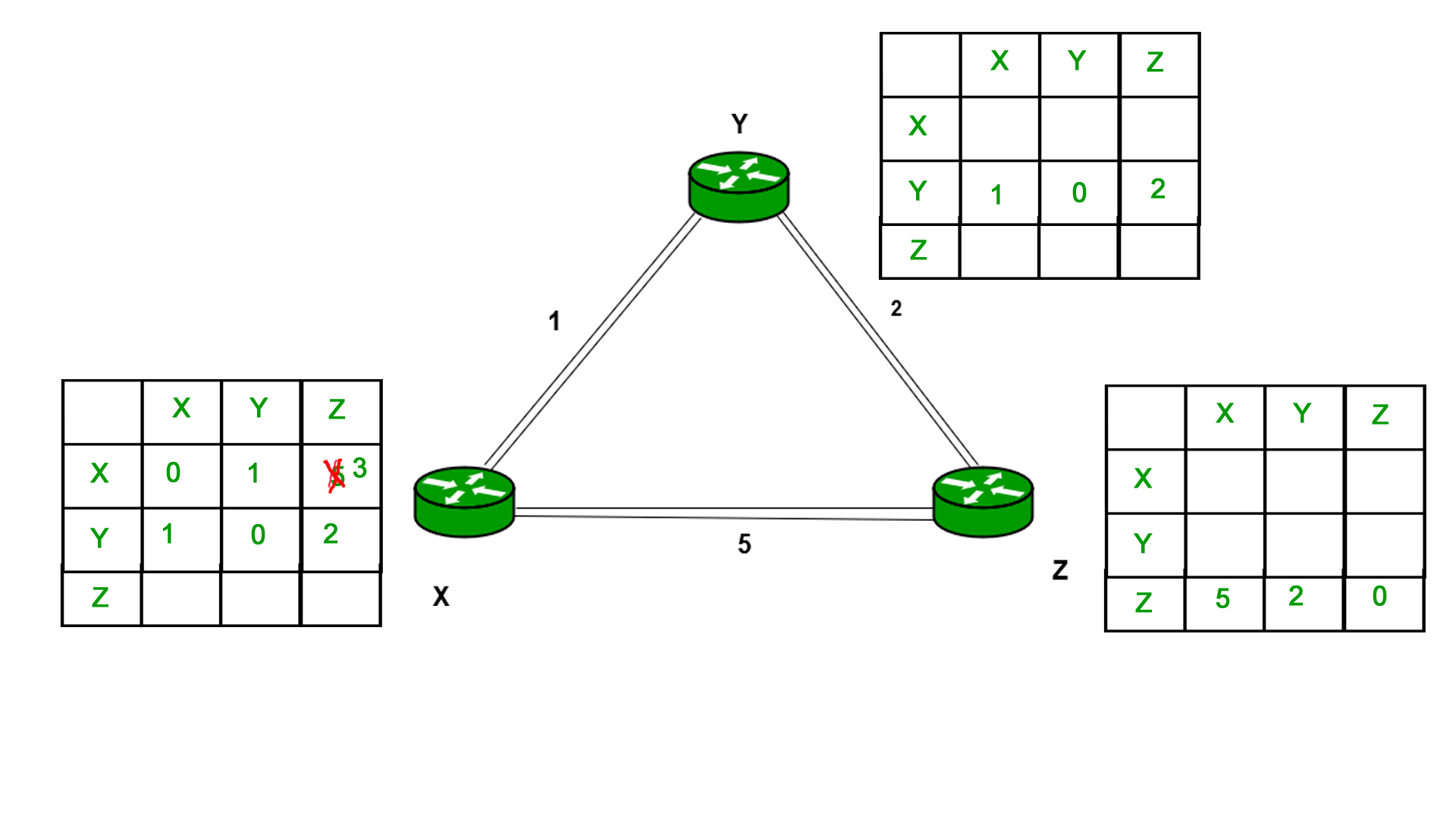# Distance Vector Routing (DVR) Protocol

• Difficulty Level : Medium
• Last Updated : 06 Nov, 2019

A distance-vector routing (DVR) protocol requires that a router inform its neighbors of topology changes periodically. Historically known as the old ARPANET routing algorithm (or known as Bellman-Ford algorithm).

Bellman Ford Basics – Each router maintains a Distance Vector table containing the distance between itself and ALL possible destination nodes. Distances,based on a chosen metric, are computed using information from the neighbors’ distance vectors.

```Information kept by DV router -
Each router has an IDAssociated with each link connected to a router,
there is a link cost (static or dynamic).Intermediate hops
Distance Vector Table Initialization -Distance to itself = 0Distance to ALL other routers = infinity number.
```

Distance Vector Algorithm –

1. A router transmits its distance vector to each of its neighbors in a routing packet.
2. Each router receives and saves the most recently received distance vector from each of its neighbors.
3. A router recalculates its distance vector when:
• It receives a distance vector from a neighbor containing different information than before.
• It discovers that a link to a neighbor has gone down.

The DV calculation is based on minimizing the cost to each destination

```Dx(y) = Estimate of least cost from x to y
C(x,v) =  Node x knows cost to each neighbor v
Dx   =  [Dx(y): y ∈ N ] = Node x maintains distance vector
Node x also maintains its neighbors' distance vectors
– For each neighbor v, x maintains Dv = [Dv(y): y ∈ N ]
```

Note –

• From time-to-time, each node sends its own distance vector estimate to neighbors.
• When a node x receives new DV estimate from any neighbor v, it saves v’s distance vector and it updates its own DV using B-F equation:
```Dx(y) = min { C(x,v) + Dv(y), Dx(y) } for each node y ∈ N
```

Example – Consider 3-routers X, Y and Z as shown in figure. Each router have their routing table. Every routing table will contain distance to the destination nodes.Consider router X , X will share it routing table to neighbors and neighbors will share it routing table to it to X and distance from node X to destination will be calculated using bellmen- ford equation.

``` Dx(y) = min { C(x,v) + Dv(y)} for each node y ∈ N
```

As we can see that distance will be less going from X to Z when Y is intermediate node(hop) so it will be update in routing table X.Similarly for Z also –Finally the routing table for all –Advantages of Distance Vector routing –

• It is simpler to configure and maintain than link state routing.
• Disadvantages of Distance Vector routing –

• It is slower to converge than link state.
• It is at risk from the count-to-infinity problem.
• It creates more traffic than link state since a hop count change must be propagated to all routers and processed on each router. Hop count updates take place on a periodic basis, even if there are no changes in the network topology, so bandwidth-wasting broadcasts still occur.
• For larger networks, distance vector routing results in larger routing tables than link state since each router must know about all other routers. This can also lead to congestion on WAN links.

Note – Distance Vector routing uses UDP(User datagram protocol) for transportation.

GATE CS Corner Questions

Practicing the following questions will help you test your knowledge. All questions have been asked in GATE in previous years or in GATE Mock Tests. It is highly recommended that you practice them.

References –

This article is contributed by Akash Sharan. If you like GeeksforGeeks and would like to contribute, you can also write an article using contribute.geeksforgeeks.org or mail your article to contribute@geeksforgeeks.org. See your article appearing on the GeeksforGeeks main page and help other Geeks.